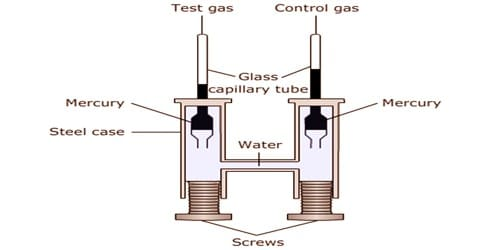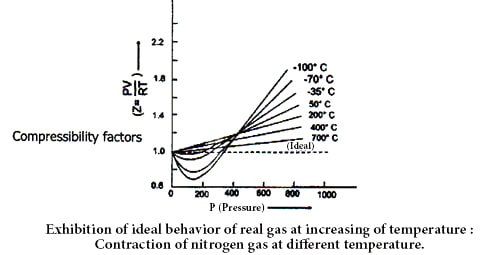# Describe Amagat’s experiment

Amagat’s experiment along with the graph:

Regnault, Andrew, Amagat and other scientists proved that real gases do not exhibit ideal behavior or nature. Ideal gas follows gas laws i.e. Boyle’s law and Charles law. In our concern of ideal gases in previous modules, we have assumed that forces between molecules forces are zero and therefore those they follow the kinetic theory of gases accurately. This is not the case with actual gases, however. According to Boyle’s law product of pressure (P) and volume is constant, at a constant temperature. Therefore, for ideal gas due to change of P, PV should be constant at a constant temperature as,  V∞ (1/P)

Amagat determined the volume of different real gases at different pressure (P) but at a fixed temperature. Then, he plotted PV against P in a graph, where he got several lines. These lines are called Amagat’s curve. For a fixed quantity of an ideal gas at a constant temperature, PV should remain constant in spite of the variation of P and consequently, the line got by plotting PV against P will be a straight line parallel to X-axis denoting pressure. It has been observed that in the case of real gases the plots of PV against P are linear but not parallel to the X-axis.

Amagat’s law or the Law of Partial Volumes of 1880 describes the behavior and properties of mixtures of ideal (as well as some cases of non-ideal) gases. Of use in chemistry and thermodynamics, Amagat’s law states that the volume Vm of a gas mixture is equivalent to the sum of volumes Vi of the K component gases if the temperature T and the pressure p stay the same: Vm(T,p) = ∑ki=1 Vi(T,p)

In the case of gases containing small molecules, like H2 and He, the value PV increases steadily with the increase of pressure from the very beginning. But for O2, N2, CO2  and other gases containing big molecules, PV at first decrease and then reaching the minimum rise with the increase of pressure.Therefore, it is undoubtedly proved that real gases do not obey gas laws i.e. they Deviate from ideal behavior. A gas which follows the gas laws exactly is known as an ideal gas and one which does not is called a real gas. But at high-temperature real gases behave like ideal gases which can be understood by the following figure.